# Greek Mathematics

## Articleby
published on 24 September 2013

The mathematicians of ancient Greece made a hugely significant contribution to world thought and all practical subjects which depend on that intellectual basis, from geometry to engineering, astronomy to design. Influenced initially by the Egyptians, Greek mathematicians would push on to make breakthroughs such as Pythagoras' theory of right-angled triangles and, by focussing on the abstract, bring clarity and precision to age-old mathematical problems. Their solutions provided the fundamental mathematical building blocks that all future mathematicians and scientists would build upon right up to the present day.

Early Influences

The birth of Greek mathematics owes its impetus to the influence of some of its neighbours, especially Egypt. During the 26th Dynasty of Egypt (c. 685–525 BCE), the ports of the Nile were opened to Greek trade for the first time and important Greek figures such as Thales and Pythagoras visited Egypt bringing with them new skills and knowledge. Ionia, in addition to Egyptian influence, was exposed to the culture and ideas of Mesopotamia through its neighbour, the kingdom of Lydia.

Some centuries later, during the Hellenistic period, Greek astronomy flourished after Alexander the Great conquered the East. The astronomical knowledge of Babylonian and Chaldean culture became available to the Greeks who profited by exploiting it systematically. This led to the advance of many Greek mathematical tools, such as the use of a numeral system with 60 as its base, which allowed the Greeks to divide circles into 360 degrees. The use of 60 as a base of a mathematical system is not a minor issue: 60 is a number that has many divisors (1, 2, 3, 4, 5, 6, 10, 12, 15, 20, 30, 60), which makes it easier to deal with calculations involving fractions.

The Egyptian influence on Greek mathematics can also be noticed in the etymology of key Greek mathematical terms. Strabo, the famous Greek geographer, explains the origin of the word geometry (which literally means “land measuring”) as follows:

The flooding of the Nile repeatedly takes away and adds soil, altering the configuration of the landscape and hiding the markers that separate one person’s land from that of someone else. Measurements have to be made over and over again, and they say that this is the origin of geometry... (Strabo, Geography 17.1.3)

Early Achievements

How is it that the Greeks managed to advance their mathematical knowledge to the point that it became superior to the Egyptians, a civilization far older? As early as 3500 BCE Egyptian (and also Babylonian) calculations were the finest in the world. Egyptians used their mathematical knowledge largely for engineering purposes; without it, building the great pyramids and other breathtaking monuments would have been impossible.

What the Greeks derived from Egyptian mathematics were mainly rules of thumb with specific applications. Egyptians knew, for example, that a triangle whose sides are in a 3:4:5 ratio is a right triangle. This was because in order to form right angles, the practical minded Egyptian land surveyors used a rope divided into twelve equal parts, forming a triangle with three parts on one side, four parts on the second side, and five parts on the remaining side. The right angle was to be found where the three-unit side joined the four-unit side. This was a very practical method to form right angles. How Egyptians came up with this method is not recorded. Neither do we have Egyptian records on further analysis related to this issue. The Egyptians were too practical to be concerned with analyzing this in detail; apparently their interest went no further than the practical application of this method. A Greek native from Ionia looked at this 3:4:5 triangle and saw in it what nobody else seemed to have noticed. His name was Pythagoras, and he stretched this 3:4:5 triangle issue to its logical limit, triggering an intellectual revolution.

Pythagoras (c.571 - c.497 BCE) was the leader and founder of a peculiar movement whose followers were known as the Pythagoreans. The members of this school were convinced that the universe could be described in terms of whole numbers: 1, 2, 3, 4, etc. Based on the 3:4:5 triangle known to the Egyptians, Pythagoras came up with a mathematical theorem that bears his name: that, in a right triangle, when the areas of the squares erected on the two smaller sides were added together, they equaled the area of the square erected on the longest side, the side opposite the right angle (the hypotenuse). It is important to note that the Greeks originally stated this theorem in terms of geometric objects instead of numbers.

Why was this theorem such an important insight? Because it shows the development of some important techniques.

1. The technique of abstraction, based on ignoring physical considerations which are seen as merely incidental. Whether it was a rope, a piece of wood or any other physical object was irrelevant. It was all about properties of “straight lines” connecting at angles, nothing more. These lines are simply mental constructs and the only entity necessary to the solution of the problem. The process of abstraction is about getting rid of all the nonessential elements and considering only what is fundamental.
2. The technique of generalization, which is about developing general principles with broad applications, rather than rules with specific use. The theorem developed by Pythagoras was true not only for the 3:4:5 triangle, but it was a principle applicable to any other right triangle, regardless of its dimensions. Furthermore, the theorem showed that a triangle is a right triangle if, and only if, the square of the longest side matches the sum of the squares of the remaining two sides: the right angle lay where the two shorter sides met.
3. The art of deductive reasoning. This is about having a set of initial general statements or premises and reaching conclusions by working out its logical implications.
4. Mathematics in the sense of demonstrative deductive arguments. By combining deductive reasoning and generalization, mathematics was no longer seen as a static set of rules but rather as a dynamic system capable of complex development.

We owe to Pythagoras, or maybe to his followers, these important Greek innovations in the field of mathematics.

The beauty and harmony that the Pythagoreans found in mathematics was so powerful that Greek science in general was eventually contaminated with a strong mathematical bias. In other words, the Greeks came to believe that deductive reasoning, which was incredibly successful in mathematics, was also the only acceptable way of obtaining knowledge in every other discipline. Observation was undervalued, deduction was made king, and Greek scientific knowledge was led up a blind alley in virtually every branch other than exact sciences. This overestimation of mathematics can be seen in a quote from Galen:

Whereas time causes grief and other emotions to alter and cease, when has the mere passage of time ever persuaded anyone that he has has enough of “twice two are four” or “all radii of a circle are equal” and made him change his mind about such beliefs and give them up? (Galen, On the Doctrines of Hippocrates and Plato 4.7.43)

The First Mathematical Crisis: The Square Root of 2

After the Pythagorean theorem was established, the following question was put forth: If we had a square with each side a unit in length, and we also had a second square with double the area of the first square, how would the side of the second square compare to the side of the first square? This is the origin of the question regarding the square root of 2.

We know today that the square root of 2 is an irrational number, which means that it cannot be expressed by any simple fraction. However, the Greeks were not aware of this, so they kept trying to solve this riddle and come up with a valid answer. Try as they might, the Pythagoreans could not solve the puzzle, and they finally faced up to the reality that no ratio of two whole numbers could express the value of the square root of 2.

The secret of irrational numbers was carefully kept by the Pythagoreans. The reason for this is that the secret created a sort of crisis in the very roots of Pythagorean beliefs. There is an interesting account (its historical accuracy is not certain) about one member of the Pythagorean circle who apparently divulged the secret to someone outside the brotherhood. The traitor was thrown into deep waters and drowned. This episode is sometimes referred to as the first martyr of science. However, we could also think about this person as one of the many martyrs of superstition, since it was not the scientific aspect of irrational numbers that was the root cause of this homicide, but rather its religious extrapolations that were seen as a threat to the foundation of Pythagorean mysticism.

The crisis of irrational numbers encouraged the creation of clever methods of approximation of the value of the square root of 2. One of the best examples of these is the method described in the following chart:

After many unsuccessful attempts in finding the value of the square root of 2, the Greeks had no choice but to accept that arithmetic could not be the basis of mathematics. They had to look somewhere else, so they looked into geometry.

The Euclidian System

Euclid (c.325-  c. 265 BCE) was an ancient Greek mathematician who lived in Alexandria. He was familiar with all of the Greek mathematical work that had preceded him, so he decided to organize all this knowledge in a single coherent work. This work has come down to us known as The Elements, and is the second best selling book of all times, surpassed only by the Bible.

The Elements is remembered mostly for its geometry. The opening of Book I begins with different definitions on basic geometry:

1. A point is that which has no part.
2. A line is breadthless length.
3. The extremities of a line are points.
4. A straight line is a line which lies evenly with the points on itself.
5. A surface is that which has length and breadth only.
6. The extremities of a surface are lines.

(Euclid, definitions 1 to 6)

There is nothing original to Euclid in the contents of The Elements (he was just a compiler). However, the order of propositions and the overall logical structure of the work is largely Euclid’s creation. It is without a doubt one of the most important and influential books ever written and a masterpiece of the Greek intellectual tradition.First English version of Euclid's Elements, 1570by Charles Thomas-Stanford (Public Domain)

From the standpoint of modern scientific knowledge, The Elements has some flaws. First, it relies solely on deduction (building conclusions on an assumed set of self-evident generalizations), not a trace of induction (starting with observations of particular facts and deriving generalizations from them) is to be found in it. Second, it follows a logical sequence by which all theorems in it could be proved through the use of theorems previously proven. This logical sequence leads us to a set of initial assumptions that cannot be proved. These assumptions are presented by Euclid as unquestionable, which means that they are so obvious that no proof is needed. An analogy of this structure would be a chain where each link is required to be connected to another link, but the initial links are simply hanging, connected nowhere.

The Delian Problem

In addition to the value of the square root of 2, there was also another famous problem that occupied the Greeks: the duplication of the cube. The legend says that:

The oracle of Apollo told the people of Delfos that, to be freed from a plague, they should build him an altar twice the size of the existing one. (Theon of Smyrna, On the Usefulness of Mathematics in McKeown)

Architects had no idea on how to solve this. The altar had the shape of a cube and the first idea that might come to one’s mind is to simply double all sides of the altar, but this leads to an altar eight times as big as the original instead of two times bigger. The right way to approach this problem is to ask: What length should each of the sides of the new altar be if we want to make the volume twice as large as the volume of the original altar? This is about determining the value of the cube root of 2, which is also an irrational number. This issue caused in geometry the same perplexity that the square root of 2 caused in arithmetic.

Greek mathematicians, including Plato, took the issue up and worked on it for centuries producing a large amount of admirable work. The central issue here is being able to determine the cube root of 2.

The Importance of Mathematical Rigour in Greeks Mathematics

The Greeks understood something that somehow eluded the Egyptians: the importance of mathematical rigour. Ancient Egyptians, for example, equated the area of a circle to the area of a square whose sides were 8/9 of the circle’s diameter. From the perspective of this calculation, the value of the mathematical constant pi is 256/81. This is a very accurate calculation (around half percent error), but mathematically incorrect. For the purposes of Egyptian engineering, however, this half percent error was not actually important, otherwise their impressive monuments would have collapsed long ago. However, ignoring this half percent error neglects a fundamental property of the true value of pi, which is that no fraction can express it. It is also an irrational number.

Egyptians also rounded up other numbers, such as the value of the square root of 2 (with the fraction 7/5). By using rounded up values, the irrational nature of these numbers was not noticed by the Egyptians. The Greeks were obsessed with mathematical rigour; for them rounding up was not good enough. They acknowledged the exactness of the mathematical language.

By not giving up in the pursuit of mathematical accuracy, the Greeks developed a mathematical knowledge that is, along with astronomy, perhaps the most admirable monument of their intellectual achievements.

## Recommended Books

•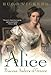Hugo Vickers St. Martin's Griffin (16 June 2003) Price: \$21.75
•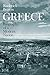Roderick Beaton University of Chicago Press (16 October 2019) Price: \$23.70
•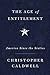Christopher Caldwell Simon & Schuster (21 January 2020) Price: \$16.80
•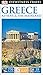DK Eyewitness DK Eyewitness Travel (20 June 2017) Price: \$17.52
•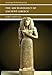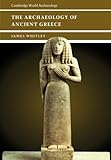James Whitley Cambridge University Press (05 November 2001) Price: \$32.21

## Cite This Work

#### APA Style

Violatti, C. (2013, September 24). Greek Mathematics. Ancient History Encyclopedia. Retrieved from https://www.ancient.eu/article/606/

#### Chicago Style

Violatti, Cristian. "Greek Mathematics." Ancient History Encyclopedia. Last modified September 24, 2013. https://www.ancient.eu/article/606/.

#### MLA Style

Violatti, Cristian. "Greek Mathematics." Ancient History Encyclopedia. Ancient History Encyclopedia, 24 Sep 2013. Web. 26 Jan 2020.Our latest articles delivered to your inbox, once a week:

Are you a...?